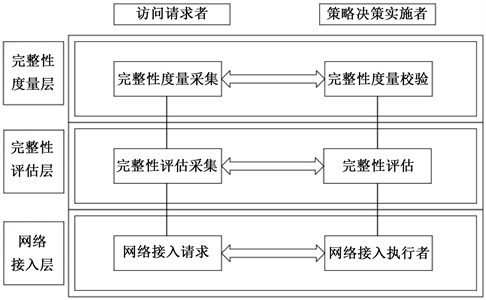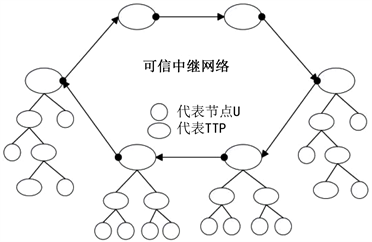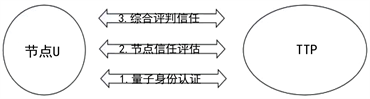# 基于量子可信中继网络的节点可信接入模型Node Trusted Access Model Based on Quantum Trusted Relay Network

• 全文下载: PDF(1282KB)    PP.611-618   DOI: 10.12677/CSA.2018.85069
• 下载量: 640  浏览量: 2,530   国家自然科学基金支持

The foundation of constructing secure communication is the establishment of trust relationship. The current quantum secure communication protocol and related technologies are based on the premise of trust. In order to solve the problem of trust in quantum communication network, this paper proposes a new scheme based on classical trusted access, based on classical trusted access, and studies the trusted access of nodes in quantum communication network. The trust evaluation system is introduced into the quantum communication network. Relying on the unique characteristics of quantum entanglement and quantum teleportation, it is proposed to borrow the quantum character for trusted access, and its process is described in detail. Finally, according to its feasibility and security, it provides a new idea for the trusted access of quantum communication network.

1. 引言

2. 可信接入的介绍

TNC规范主要可分为两大部分，一部分是对身份的证明，另一部分是对完整性的度量。在身份的证明过程中通过对用户及平台的身份认证来完成身份的证明；在完整性的度量过程中对病毒库完整性，授权软件应用版本，身份归属，安全策略等因素进行检查来确定其结果。其中对身份和完整性的证明过程中均使用到了基于离散数学的公钥密码学。安全性由复杂的数学运算来保证。在量子网络中可以利用量Figure 1. TNC framework

3. 接入流程

3.1. 量子身份认证Figure 2. Trusted relay networkFigure 3. Flow chart

ID的第i位为0时，生成第i个光子 $|0〉$$|\text{1}〉$ ；当ID的第i位为1时，生成第i个光子 $|+〉$$|-〉$ 。再根据上述规则制备若干位诱惑光子序列S’并插入到光子序列S中。双方约定根据ID的第i位信息来对测量基进行选取。其中当ID的第i位为0时，选取Z基；其中当ID的第i位为1时，选取X基。节点u在收到TTP发送的光子序列S后，TTP向节点u公布光子序列S中诱惑粒子的位置。根据TTP公布的诱惑粒子位置，节点u将S’提取出来，然后根据相互约定的规则选取测量基对诱惑光子序列S’和光子序列S按顺序进行测量。最后将测量结果进行公布。如果诱惑粒子的测量结果没有超过窃听检测的阈值范围，TTP根据光子序列S的测量结果来完成对节点u的身份认证。根据上述规则，TTP对节点u的认证过程如图4所示。

3.2. 基于可信中继网络的节点信任评估

TTP与用户 ${u}_{i}\left(i=1,2,\cdots ,n\right)$ 之间共享一对Bell粒子，将其中粒子1归节点 ${u}_{i}\left(i=1,2,\cdots ,n\right)$ 所有，粒子2归TTP，所有四种Bell态表示如下：Figure 4. Node U and TTP for quantum authentication

${|{\varphi }^{+}〉}_{12}=\frac{1}{\sqrt{2}}{\left(|00〉+|11〉\right)}_{12}$ ; ${|{\varphi }^{-}〉}_{12}=\frac{1}{\sqrt{2}}{\left(|00〉-|11〉\right)}_{12}$ ;

${|{\psi }^{+}〉}_{12}=\frac{1}{\sqrt{2}}{\left(|01〉+|10〉\right)}_{12}$ ; ${|{\psi }^{-}〉}_{12}=\frac{1}{\sqrt{2}}{\left(|01〉-|10〉\right)}_{12}$

${u}_{i}$ 将自身的信任属性制备成量子态 ${|\alpha 〉}_{U}=\mathrm{cos}\theta |0〉+\mathrm{sin}\theta |1〉$ (即要隐形传态的量子态)，借助于量子信道隐形传态给TTP。具体过程如下：

1)  对量子态 ${|\alpha 〉}_{U}$ 和Bell态进行直积操作。

${|{\varphi }^{+}〉}_{12}=\frac{1}{\sqrt{2}}{\left(|00〉+|11〉\right)}_{12}$ 的粒子1和粒子2进行“ $\otimes$ ”运算，得到一个三粒子态 ${|\phi 〉}_{U}$ 。过程如下。

${|\phi 〉}_{U}={|\alpha 〉}_{u}\otimes {|{\varphi }^{+}〉}_{12}=\left(\mathrm{cos}\theta |0〉+\mathrm{sin}\theta |1〉\right)\otimes \frac{1}{\sqrt{2}}{\left(|00〉+|11〉\right)}_{12}$

2) ${u}_{i}$ 对粒子1和量子态进行测量。判断其结果处于四种Bell态中的哪一种。此处对量子信息进行编码。用经典信息二进制00、01、10和11分别代表 ${u}_{i}$ 的测量结果 ${|{\varphi }^{+}〉}_{U1}$${|{\varphi }^{-}〉}_{U1}$${|{\psi }^{+}〉}_{U1}$${|{\psi }^{-}〉}_{U1}$ 四个状态，并将结果(经典信息)发送给TTP。此处将 ${|\phi 〉}_{U}$ 按对应的四个Bell态展开得到：

$\begin{array}{l}{|\phi 〉}_{U}=1/2\left[{|{\varphi }^{+}〉}_{U1}\left(\mathrm{cos}\theta {|0〉}_{2}+\mathrm{sin}\theta {|1〉}_{2}\right)+{|{\varphi }^{-}〉}_{U1}\left(\mathrm{cos}\theta {|0〉}_{2}-\mathrm{sin}\theta {|1〉}_{2}\right)\\ \text{\hspace{0.17em}}\text{\hspace{0.17em}}\text{\hspace{0.17em}}\text{\hspace{0.17em}}\text{\hspace{0.17em}}\text{\hspace{0.17em}}\text{\hspace{0.17em}}\text{\hspace{0.17em}}\text{\hspace{0.17em}}\text{ }\text{ }+{|{\psi }^{+}〉}_{U1}\left(\mathrm{cos}\theta {|1〉}_{2}+\mathrm{sin}\theta {|0〉}_{2}\right)+{|{\psi }^{-}〉}_{U1}\left(\mathrm{cos}\theta {|1〉}_{2}-\mathrm{sin}\theta {|0〉}_{2}\right)\right]\end{array}$

3) TTP根据收到的测量结果，对他拥有的粒子2做对应的操作，即可恢复出 ${u}_{i}$ 信任值的原始量子态 ${|\alpha 〉}_{U}=\mathrm{cos}\theta |0〉+\mathrm{sin}\theta |1〉$ 。并规定当TTP收到 ${u}_{i}$ 发送的经典信息00、01、10、11时，做相对应酉变换，即可得到 ${u}_{i}$ 信任值的原始量子态 ${|\alpha 〉}_{U}$ ，对应关系如表1所示。

4) TTP根据恢复出的 ${|\alpha 〉}_{U}=\mathrm{cos}\theta |0〉+\mathrm{sin}\theta |1〉$ ，计算出 ${u}_{i}$ 的信任值，并根据计算出的信任值评判是否信任 ${u}_{i}$ ，同时将 ${u}_{i}$ 的信任值保存(更新)在TTP所属的本地数据库中。

3.3. 模糊综合评判信任模型Table 1. The unitary transformation of classical information

$\left(\begin{array}{cccc}0.3& 0.7& 0& 0\\ 0.4& 0.5& 0.1& 0\\ 0.5& 0.5& 0& 0\\ 0.2& 0.3& 0.45& 0.05\\ 0.3& 0.3& 0.25& 0.15\end{array}\right)$

TTP通过对算子的选取来计算出评价向量 $I=W\cdot R=\left(0.33，0.56,0.09,0.02\right)$ 。最后通过评价集与评价向量进行量化，将节点u的信任值计算出。 $D\cdot {I}^{\text{T}}=0.92$ 。TTP根据自身的接入标准设置一个阈值用来判断节点是否接入。假设该阈值为0.90，则节点u接入成功。

4. 安全性分析Table 2. Each attribute of the node corresponds to the evaluation set

4.1. 身份认证的安全性

4.2. 信任评估的安全性

5. 小节

  Bennett, C.H. and Brassard, G. (1984) Quantum Cryptography: Public Key Distribution and Coin Tossing. Proceedings of the IEEE International Conference on Computers, Systems and Signal Processing, Bangalore, 175-179.  Ekert, A.K. (1991) Quantum Cryptography Based on Bell’s Theorem. Physical Review Letters, 67, 661-663. https://doi.org/10.1103/PhysRevLett.67.661  徐兵杰, 刘文林, 毛钧庆, 等. 量子通信技术发展现状及面临的问题研究[J]. 通信技术, 2014, 47(5): 463-468.  Sasaki, M., Fujiwara, M., Ishizuka, H., et al. (2011) Field Test of Quantum Key Distribu-tion in the Tokyo QKD Network. Optics Express, 19, 10387-10409. https://doi.org/10.1364/OE.19.010387  Zhang, H., Chen, L. and Zhang, L. (2010) Research on Trusted Network Connection. Jisuanji Xuebao (Chinese Journal of Computers), 33, 706-717. https://doi.org/10.3724/SP.J.1016.2010.00706  Zhang, H.G., Han, W.B., Lai, X.J., et al. (2015) Survey on Cy-berspace Security. Science China Information Sciences, 58, 1-43. https://doi.org/10.1007/s11432-015-5433-4  Arrazola, J.M. and Lütkenhaus, N. (2014) Quantum Communication with Coherent States and Linear Optics. Physical Review A, 90, Article ID: 042335. https://doi.org/10.1103/PhysRevA.90.042335  Shi, R.H., Huang, L.S., Yang, W., et al. (2010) Quantum Secret Sharing between Multiparty and Multiparty with Bell States and Bell Measurements. Science China Physics, Mechanics & Astronomy, 53, 2238-2244. https://doi.org/10.1007/s11433-010-4181-0  Sun, Y., Xu, S.W., Chen, X.B., et al. (2013) Expansible Quantum Secret Shar-ing Network. Quantum Information Processing, 12, 2877-2888. https://doi.org/10.1007/s11128-013-0570-4  张仕斌, 许春香. 基于云模型的信任评估方法研究[J]. 计算机学报, 2013, 36(2): 422-431.  Chang, Y.J., Tsai, C.W. and Hwang, T. (2013) Multi-User Private Comparison Protocol Using GHZ Class States. Quantum Information Processing, 12, 1077-1088. https://doi.org/10.1007/s11128-012-0454-z